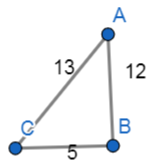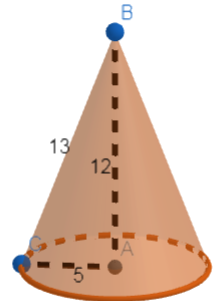Courses
Courses for Kids
Free study material
Free LIVE classes
More# A right triangle ABC with sides 5cm, 12cm, and 13cm is revolved about the side 5cm. Find the volume of the solid so obtained. Also find the ratio of volume of two solids obtained.

Last updated date: 21st Mar 2023
Total views: 305.4k
Views today: 4.83kVerified
305.4k+ views
Hint: In this question, first draw the diagram it will give a clear idea about what we have to find. Now, draw the shape that would form on revolving the triangle and then find the volume of that shape formed.

Let us first draw the right angle triangle ABC.Now, if we rotate this triangle about the side of 5cm the solid formed will be:CONE: A cone is a three-dimensional geometric shape that tapers smoothly from a flat base to a point called the vertex.

A cone is formed by a set of line segments, half-lines, or lines connecting a common point, the vertex, to all the points on a base that is in a plane that does not contain the vertex.
It forms a cone of radius 5cm and height 12 cm.
Let us now look at the formula for volume of a cone.
Volume of a right circular cone $=\dfrac{\pi }{3}{{r}^{2}}h$
Where, r is the radius of the cone.
h is the height of the cone.
Now, by substituting the respective values in the above formula we get,
\begin{align} & r=5 \\ & h=12 \\ \end{align}
Let us assume the volume of the cone as V.
$V=\dfrac{\pi }{3}{{r}^{2}}h$
$\Rightarrow V=\dfrac{\pi }{3}{{\left( 5 \right)}^{2}}\left( 12 \right)$
Now, on simplification we get,
\begin{align} & \Rightarrow V=\dfrac{\pi }{3}\times 25\times 12 \\ & \Rightarrow V=\pi \times 25\times 4 \\ & \Rightarrow V=100\pi \\ & \Rightarrow V=100\times 3.14\text{ }\left[ \because \pi =3.14 \right] \\ & \therefore V=314c{{m}^{3}} \\ \end{align}
Hence, the volume of the solid obtained is 314cm3 .
If it is revolved about the side 12 cm then we get,
\begin{align} & r=12 \\ & h=5 \\ \end{align}
By substituting these in the formula of volume of a cone we get,
$V=\dfrac{\pi }{3}{{r}^{2}}h$
$\Rightarrow V=\dfrac{\pi }{3}{{\left( 12 \right)}^{2}}\left( 5 \right)$
$\Rightarrow V=\dfrac{\pi }{3}\times 144\times 5$
$\Rightarrow V=\pi \times 48\times 5$

\begin{align} & \Rightarrow V=240\pi \\ & \Rightarrow V=240\times 3.14\text{ }\left[ \because \pi =3.14 \right] \\ & \therefore V=753.6c{{m}^{3}} \\ \end{align}
Now, the ratio of the two solids so obtained is:
\begin{align} & \Rightarrow \dfrac{100\pi }{240\pi } \\ & \Rightarrow \dfrac{100}{240} \\ & \Rightarrow \dfrac{5}{12} \\ \end{align}
Hence, the ratio of the two solids formed is $\dfrac{5}{12}$.

Note: It is important to note what would be the solid formed on revolving on a right angled triangle and in doing so we need to keep a note about which side it is being rotated. So, the values of radius and height changes accordingly.

We considered 5cm radius when it revolved about 5cm and then later we considered that it revolved around 12cm. But, we cannot consider to revolve it about 13cm side because if so it does not form a right circular cone.

While substituting the respective values in the given formula care should be taken because change in the substitution of radius and height in the other way causes error in the total result.